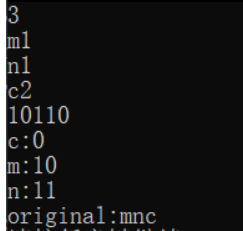yuanwei_jiuyin 2022-03-06 22:51 采纳率: 0%

# 7-2 哈夫曼编码译码 (25 分) 编写一个哈夫曼编码译码程序。

3
m1
n1
c2
10110

c:0
m:10
n:11
original:mnc

• 写回答

#### 1条回答默认 最新

•关注``````#include <iostream>
#include <stdio.h>
#include<stdlib.h>
#include<string.h>
#include<vector>
#include<map>
#include<math.h>
#include <algorithm>
using namespace std;

/**
*  树结点
*/
struct TreeNode{
double data; //权值
char ch;//字符
struct TreeNode *left;//左孩子,默认指向空
struct TreeNode *right;//右孩子
} Node;
map<string,char> m;  //定义一个map

/*********定义函数***************/
bool cmp(struct TreeNode a,struct TreeNode b);
TreeNode* createTree(TreeNode *v,int n);
void printCode(struct TreeNode *root,char code[],int location);
void f_decode(char decoding[],int len);
/****************************/
int main()
{

int n;
scanf("%d",&n);
getchar();//吸收回车字符
//定义n个结点，使用vector存放
TreeNode v[2*n-1];
//输入n个结点
for(int i=0;i<n;i++){
scanf("%c%lf",&v[i].ch,&v[i].data);
v[i].left=NULL;//默认指向NULL
v[i].right=NULL;
getchar();
}
//输入需进行译码的串
char decoding;
scanf("%s",decoding);
int len = strlen(decoding);//统计长度

//根节点
TreeNode* root=(TreeNode*)malloc(sizeof(TreeNode));
//生成树
root=createTree(v,n);
//cout<<"根节点权值为："<<root->data<<endl;

char code={0};//存储字符的编码
//先序顺序输出所有字符的编码
printCode(root,code,0);

//解码
/*
for(int i=0;i<5;i++){//打印输入的一串字符
printf("%c",decoding[i]);
}
printf("\n");

cout<<"输出map："<<endl;
for(auto  &it:m){//打印map中的数据
string str=it.first;
cout<<str<<":"<<it.second<<endl;
}
printf("\n字符串的长度为：%d\n",len);
*/
f_decode(decoding,len);

system("pause");
return 0;
}
/**
*解码 ，码——>原文
*/
void f_decode(char decoding[],int len)
{
string str;
int i=0;
printf("original:");
for(i=0;i<len;i++)
{
str+=decoding[i];//拼接key
if(m.find(str)!=m.end()){//找到了key，输出
printf("%c",m[str]);
str="";//清空key，重新拼接
}
else
{
//找不到key，什么也不用做，继续拼接，这个可以去掉
}
}
printf("\n");
}

/**
* 先序顺序输出所有字符的编码
*/
void printCode(TreeNode *root,char code[],int location){
if (root == NULL) {
return;
}
if(root->ch!='-')//说明已经遍历到叶子节点，输出序列
{
printf("%c:",root->ch);
string key="";
for(int i=0;i<location;i++){//输出记录的长度。
printf("%d",code[i]);
key+=to_string(code[i]);//to_string()将参数转为string类型
}
printf("\n");
//cout<<"key:"<<key<<","<<"value："<<root->ch<<endl;
m.insert(make_pair(key, root->ch));//将值以键值对的形式插入到map中
}
//该节点是左孩子,路径设0
code[location]=0;
location++;//下一个位置给下一个节点用
printCode(root->left,code,location); //递归该节点。
location--;//该节点递归完成。取消该节点的记录。

//右孩子,1
code[location]=1;
location++;
printCode(root->right,code,location);
location--;
}

/**
* 创建树
*/
TreeNode* createTree(TreeNode *v,int n){

int i=0,index=n-1; //i为0 ，从位置0开始合成。index是生成的结点该放的地方
while(i<2*n-1) //当i走到最后一个结点，无需再构建，也可以使用index控制
{
//1.先排序
sort(v+i,v+index,cmp);  //只对范围内的排序。随两个索引变化，排序的范围也变化
//2.合并:拿出排好序序列中的前两个结点。
TreeNode* node1 = &v[i++];//TreeNode* node1 = &v[i];i++;
TreeNode* node2 = &v[i++];

//3.生成新节点，给结点赋值
TreeNode newNode=v[index];
newNode.data=node1->data+node2->data; //权值相加
newNode.ch='-';//随意设置，表示其为中间结点。
newNode.left=node1;//设置左右孩子
newNode.right=node2;

//4.新节点放入到序列中
v[++index]=newNode;
}
return &v[index-1]; //最后一个为根节点
}

/**
* 排序规则
*/
bool cmp(TreeNode a, TreeNode b)
{
return a.data < b.data; //根据权值升序排序
}

``````
评论

#### 悬赏问题

• ¥15 求制作一个个人网页，
• ¥15 寻涂色内存脚本作者有项目有市场有资源.却技术
• ¥15 蓝桥杯c51单片机问题
• ¥15 ajax跨域问题请求修改代码
• ¥15 python matplotlib
• ¥15 短信测压+语音，有偿，必须用Python
• ¥20 COCOS2DX的protobuf协议注册函数问题
• ¥15 (标签-Pytorch|关键词-Stream)
• ¥15 求深圳2019年开放数据应用创新大赛的营运车辆数据！
• ¥15 软件UI界面绘制折线图